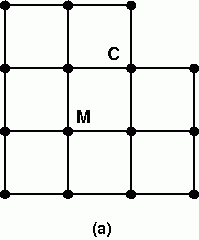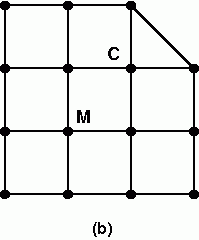## 2008 Qualifying Quiz

You may also be interested in the printable (PDF) format.

1. There are 26 students at Logic Camp; 18 of them always tell the truth and the other 8 always lie. Of course, everyone at the camp knows who is who. One day, n campers are sitting in a circle. Each of them says: "Exactly one of my two neighbors is a liar." For what values of n > 2 is this possible? (See note 1.)

2. Several Mathcampers are hiking on Mount St. Helens when it suddenly starts raining. Luckily, some of them brought umbrellas, so they huddle in groups, with one umbrella per group. Within each group, the space under the umbrella might not be split equally. Any person who ends up with less than a quarter of an umbrella will get soaked. If two thirds of the groups are larger than 5 people, prove that at least a quarter of the hikers will get soaked. (See Note 2.)

3. The increasing sequence 1,3,4,9,10,12,13,... consists of all those positive integers which are powers of 3 or sums of distinct powers of 3. Of the first googol (10100) terms in the sequence, how many are powers of 3?

4. Every Spanish citizen is assigned an ID that consists of eight digits (0–9) and one letter. The letter is not assigned randomly: only 23 letters are used, which correspond to the 23 possible remainders on dividing the eight-digit number by 23. The letter acts as a "check symbol": if somebody makes a mistake when entering the number, chances are that the resulting ID will not be valid (the letter will be wrong) and the error will be detected right away.

1. Show that if one of the eight digits is entered incorrectly (e.g., 12349678 in place of 12345678) or if two digits are mistakenly swapped (e.g., 12845673 in place of 12345678), the letter will always be wrong.
2. 23 is called the "modulus" of the check symbol. The Spanish alphabet has 27 letters, so the check symbol could have been based on any modulus up to 27. Show that 23 is the largest possible modulus less than or equal to 27 for which both of the conditions in (a) hold. Find another such modulus, and show that it works as well.

5. The diagonals of quadrilateral ABCD intersect in point E. Given that |AB|=|CE|, |BE|=|AD|, and ∠AED = ∠BAD, determine the ratio |BC| / |AD|. (|XY| means the length of the segment from point X to point Y.)

6. A cat is trying to catch a mouse in each of the labyrinths (a), (b), and (c):The cat starts at vertex C and the mouse at vertex M. The cat and the mouse take turns moving, with the cat moving first; each move is from a vertex to an adjacent vertex. If the cat ever gets to the same vertex as the mouse, it catches the mouse. In which (if any) of the three labyrinths can the cat catch the mouse, assuming the mouse makes no stupid moves? (A "stupid move" is a move after which the cat has a strategy that guarantees it can catch the mouse, where it didn't have one before.)

7. Consider an infinite chessboard, with numbers in each square. Initially all of the numbers are 0, except for a single 1 on a black square. Go through a series of steps: at every step, replace each number with the sum of its four neighbors (all numbers get replaced simultaneously).

1. After n steps, what is the sum of all the numbers on the board? What number is in the position of the original 1?
2. Give as precise a description as you can of the arrangement of numbers on the board after n steps. (Even if you know the answer, you may find it hard to state clearly. Do your best: this is part of the challenge of the problem.)

8. Amy, Brian, and CoScott are playing a game, with Dana as the judge. Dana puts eight stickers (four red and four blue) in a bowl, then draws two at random for each player and sticks them to their foreheads. Each player can see both of the other players' stickers, but not his or her own or the two left in the bowl. Dana then asks Amy whether she knows the colors of her stickers. If Amy does not, Dana asks Brian whether he knows his colors, then CoScott, then Amy again, then Brian again, and so on. The game ends if one of the players can logically deduce his or her colors. What is the probability that the game ends on one of Amy's turns? Brian's? CoScott's? What is the probability that it never ends at all?

9. You are in charge of a project to design a card-shuffling machine for a deck of n cards, initially ordered from 1 to n. The machine will repeatedly take the top card and re-insert it randomly into the deck, so that all positions for the inserted card are equally likely (including the top and bottom). The machine can keep track of where each insertion happens, so that at every point the current order of the cards is known. Your job is to tell the machine in advance when it should stop shuffling. In your instructions, you can refer to the current state of the deck (e.g. "Go until card 2 is on the bottom, then go until card n-1 is third from the top, then shuffle 5 more times, then stop"). You want to program the machine so that when it stops, all permutations of the deck will be equally likely. What instructions should you give to the machine? (Be sure to prove that these instructions really do lead to all permutations being equally likely.) With these instructions, how many insertions will it take, on average, until the machine stops?

10. Asaf is investigating rectangles drawn in the plane such that every vertex has integer coordinates. (The sides of the rectangle need not be parallel to the coordinate axes.) He considers two rectangles to be the same if one can be obtained by rotating and/or translating the other.

1. How many distinct rectangles can Asaf draw with area 100?
2. How many distinct rectangles can Asaf draw with a given integer area A? (See Note 3.)

### Notes

1. Whenever a math problem asks you for all numbers that satisfy a certain property, your task is actually three-fold: you need to come up with the correct list of numbers, show that all the numbers on your list do satisfy the property, and show that no other numbers work.
2. The main challenge in this problem is in the writing. Phrase your argument carefully, with no unwarranted assumptions and no unnecessary information. Make sure every step in your reasoning is clear, relevant, and 100% correct.
3. The solution to Part (b) uses a fact from fairly advanced number theory. If you don't know it, you may need to look it up in a book or online when you get to the point where you need it. Remember that the quiz rules do allow you to look up standard techniques and theorems, provided you reference anything you use.

Problems 1 and 7 copyright Mark Krusemeyer.